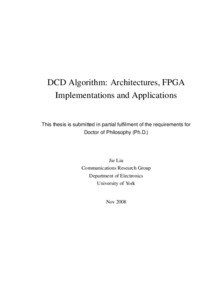# DCD Algorithm: Architectures, FPGA Implementations and Applications

Liu, Jie (2008) DCD Algorithm: Architectures, FPGA Implementations and Applications. PhD thesis, University of York.Preview
Text (PhD thesis by Jie Liu, Electronics Department, 2008)
PhD_thesis_Jie_Liu.pdf
Available under License Creative Commons Attribution-Noncommercial-No Derivative Works 2.0 UK: England & Wales.

Download (1858Kb)

## Abstract

In areas of signal processing and communications such as antenna array beamforming, adaptive filtering, multi-user and multiple-input multiple-output (MIMO) detection, channel estimation and equalization, echo and interference cancellation and others, solving linear systems of equations often provides an optimal performance. However, this is also a very complicated operation that designers try to avoid by proposing different sub-optimal solutions. The dichotomous coordinate descent (DCD) algorithm allows linear systems of equations to be solved with high computational efficiency. It is a multiplication-free and division-free technique and, therefore, it is well suited for hardware implementation. In this thesis, we present architectures and field-programmable gate array (FPGA) implementations of two variants of the DCD algorithm, known as the cyclic and leading DCD algorithms, for real-valued and complex-valued systems. For each of these techniques, we present architectures and implementations with different degree of parallelism. The proposed architectures allow a trade-off between FPGA resources and the computation time. The fixed-point implementations provide an accuracy performance which is very close to the performance of floating-point counterparts. We also show applications of the designs to complex division, antenna array beamforming and adaptive filtering. The DCD-based complex divider is based on the idea that the complex division can be viewed as a problem of finding the solution of a 2x2 real-valued system of linear equations, which is solved using the DCD algorithm. Therefore, the new divider uses no multiplication and division. Comparing with the classical complex divider, the DCD-based complex divider requires significantly smaller chip area. A DCD-based minimum variance distortionless response (MVDR) beamformer employs the DCD algorithm for multiplication-free finding the antenna array weights. An FPGA implementation of the proposed DCD-MVDR beamformer requires a chip area much smaller and throughput much higher than that achieved with other implementations. The performance of the fixed-point implementation is very close to that of floating-point implementation of the MVDR beamformer using direct matrix inversion. When incorporating the DCD algorithm in recursive least squares (RLS) adaptive filter, a new efficient technique, named as the RLS-DCD algorithm, is derived. The RLS-DCD algorithm expresses the RLS adaptive filtering problem in terms of auxiliary normal equations with respect to increments of the filter weights. The normal equations are approximately solved by using the DCD iterations. The RLS-DCD algorithm is well-suited to hardware implementation and its complexity is as low as O(N2) operations per sample in a general case and O(N) operations per sample for transversal RLS adaptive filters. The performance of the RLS-DCD algorithm, including both fixed-point and floating-point implementations, can be made arbitrarily close to that of the floating-point classical RLS algorithm. Furthermore, a new dynamically regularized RLS-DCD algorithm is also proposed to reduce the complexity of the regularized RLS problem from O(N^3) to O(N^2) in a general case and to O(N) for transversal adaptive filters. This dynamically regularized RLS-DCD algorithm is simple for finite precision implementation and requires small chip resources.

Item Type: Thesis (PhD) The University of York > Electronics (York) uk.bl.ethos.488739 Dr Yuriy Zakharov 02 Sep 2011 15:28 08 Aug 2013 08:46 http://etheses.whiterose.ac.uk/id/eprint/1629

You do not need to contact us to get a copy of this thesis. Please use the 'Download' link(s) above to get a copy.
You can contact us about this thesis. If you need to make a general enquiry, please see the Contact us page.